JEE  >  JEE Main Mock Test 3

# JEE Main Mock Test 3

Test Description

## 90 Questions MCQ Test | JEE Main Mock Test 3

JEE Main Mock Test 3 for JEE 2023 is part of JEE preparation. The JEE Main Mock Test 3 questions and answers have been prepared according to the JEE exam syllabus.The JEE Main Mock Test 3 MCQs are made for JEE 2023 Exam. Find important definitions, questions, notes, meanings, examples, exercises, MCQs and online tests for JEE Main Mock Test 3 below.
Solutions of JEE Main Mock Test 3 questions in English are available as part of our course for JEE & JEE Main Mock Test 3 solutions in Hindi for JEE course. Download more important topics, notes, lectures and mock test series for JEE Exam by signing up for free. Attempt JEE Main Mock Test 3 | 90 questions in 180 minutes | Mock test for JEE preparation | Free important questions MCQ to study for JEE Exam | Download free PDF with solutions
 1 Crore+ students have signed up on EduRev. Have you?
JEE Main Mock Test 3 - Question 1

### The total length of a sonometer wire between fixed ends is 110 cm. Two bridges are placed to divide the length of wire in ratio 6 : 3 : 2. The tension in the wire is 400 N and the mass per unit length is 0.01 kg/m. What is the minimum common frequency with which three parts can vibrate?

JEE Main Mock Test 3 - Question 2

### Two factories are sounding their sirens at 800 Hz. A man goes from one factory to other at a speed of 2 m/s. The velocity of sound is 320 m/s. The number of beats heard by the person in one second will be

JEE Main Mock Test 3 - Question 3

### Interference pattern is observed at ‘P’ due to superimposition of two rays coming out from a source ‘S’ as shown in the figure. The value of l for which maxima is obtained at ‘P’ is ( R is perfect reflection surface):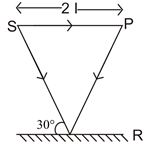JEE Main Mock Test 3 - Question 4

Two bodies of masses 1 kg and 4 kg are connected to a vertical spring, as shown in the figure. The smaller mass executes simple harmonic motion of angular frequency 25 rad/s, and amplitude 1.6 cm while the bigger mass remains stationary on the ground. The maximum force exerted by the system on the floor is (take g = 10 ms-2).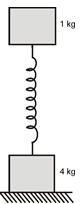JEE Main Mock Test 3 - Question 5

An ideal gas enclosed in a vertical cylindrical container supports a freely moving piston of mass M. The piston and cylinder have equal cross sectional area A. When the piston is in equilibrium, the volume of the gas is V0 and its pressure is P0. The piston is slightly displaced from the equilibrium position and released. Assuming that the system is completely isolated from its surrounding, the piston executes a simple harmonic motion with frequency:

JEE Main Mock Test 3 - Question 6

A pendulum made of a uniform wire of cross sectional area A has time period T. When an additional mass M is added to its bob, the time period changes to TM. If the Young’s modulus of the material of the wire is Y then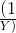is equal to : (g-gravitational acceleration)

JEE Main Mock Test 3 - Question 7

Consider an ideal gas confined in an isolated closed chamber. As the gas undergoes an adiabatic expansion the average time of collision between molecules increases as Vq, where V is the volume of the gas. The value of q is :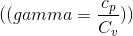JEE Main Mock Test 3 - Question 8

An ideal mono-atomic gas is confined in a cylinder by a spring loaded piston of cross section 0.8 × 10-2m2. Initially the gas is at 300 K and occupies a volume of 2.4 × 10-3m3 and the spring is in its relaxed state as shown in figure. The gas is heated by a small heater until the piston moves out slowly by 0.1 m. The force constant of the spring is 8000 N/m and the atmospheric pressure is 1.0 × 105 N/m2. The cylinder and the piston are thermally insulated. The piston and the spring are massless and there is no friction between the piston and the cylinder. The final temperature of the gas will be ( Neglect the heat loss through the lead wires of the heater. The heat capacity of the heater coil is also negligible)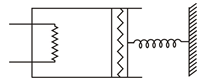JEE Main Mock Test 3 - Question 9

The temperature-entropy diagram of a reversible engine cycle is given in the figure. Its efficiency is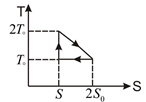JEE Main Mock Test 3 - Question 10

A black coloured solid sphere of radius R and mass M is inside a cavity with vacuum inside. The walls of the cavity are maintained at temperature T0. The initial temperature of sphere is 3T0. If the specific heat of the material of the sphere varies as αT3 with the temperature T of the sphere, where a is a constant, then the time taken for the sphere to cool down to temperature 2T0 will be (σ is Stafan-Boltzmann constant )

JEE Main Mock Test 3 - Question 11

A thin tube sealed at both ends is 100 cm long. It lies horizontally, the middle 20 cm containing mercury and two equal ends containing air at standard atmospheric pressure. If the tube is now turned to a vertical position, by what amount will the mercury be displaced? (Given: cross section of the tube can be assumed to be uniform)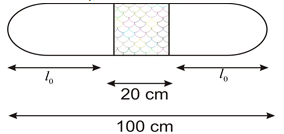JEE Main Mock Test 3 - Question 12

A thin uniform annular disc (see figure) of mass M has outer radius 4R and inner radius 3R. The work required to take a unit mass from point P on its axis to infinity is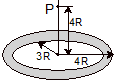JEE Main Mock Test 3 - Question 13

A small particle of mass m is projected at an angle θ with the x-axis with an initial velocity v0 in the x-y plane as shown in the figure. At a time t <, the angular momentum of the particle is

JEE Main Mock Test 3 - Question 14

Consider a cylinder of mass M resting on a rough horizontal rough that it pulled out from under it with acceleration ‘a’ perpendicular to the axis of cylinder. What is Ffriction at point P? It is assumed that the cylinder does not slip.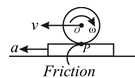JEE Main Mock Test 3 - Question 15

A mass m moves with a velocity u and collides elastically with another identical mass at rest. After collision the first mass moves with celcity in a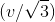direction perpendicular to the initial direction of motion. Find the speed of the second mass after collision.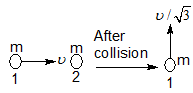JEE Main Mock Test 3 - Question 16

The acceleration experienced by a moving motor boat, after its engine is cut off, is given by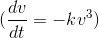.Where k is constant. If v0 is the magnitude of velocity at cut-off, the magnitude of the velocity at a time t after the cut off is

JEE Main Mock Test 3 - Question 17

The distance between the object and its doubly magnified real image by a concave mirror of focal length f is

JEE Main Mock Test 3 - Question 18

The diameter of objective lens of microscope makes an angle β at the focus of the microscope. Further, the medium between the object and the lens is an oil of refractive index n. Then the resolving power of microscope.

JEE Main Mock Test 3 - Question 19

In a Young’s double slit experiment, the distance between the two identical slits is 6.1 times larger than the slit width. Then the number of intensity maxima observed within the central maximum of the single slit diffraction pattern is:

JEE Main Mock Test 3 - Question 20

An isolated sphere S of radius R carries an electric charge. S is momentarily connected to a distant uncharged sphere T which has a radius r. The ratio of surface charge density of S to that of T is

JEE Main Mock Test 3 - Question 21

In post office box, the graph of galvanometer deflection versus resistance R ( pulled out of resistance box) for the ratio 100:1 is given as shown ( due to unsuitable values of R, galvanometer shows deflection).The two consecutive values of R are shown in the figure.The value of unknown resistance would be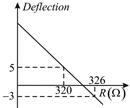JEE Main Mock Test 3 - Question 22

A sphere encloses an electric dipole within it. The total flux across the sphere is

JEE Main Mock Test 3 - Question 23

The output of a 2 input NAND gate is fed to a NOT gate. The Truth table for the output of the combination for all possible input of A and B is

JEE Main Mock Test 3 - Question 24

A zener diode is operating in its normal region i.e., the breakdown region for which the circuit diagram is as shown in the figure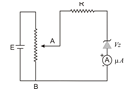Here, Take VZ = 7V and R = 10 W. For potential difference equal to 8V across AB, what is the current through microammeter?

JEE Main Mock Test 3 - Question 25

Voltage with appreciable ripple expressed as V = V1+V2 cos wt is applied to a resistor R. The average amount of heat generated per second is

JEE Main Mock Test 3 - Question 26

What is the electric potential at the centre of the square?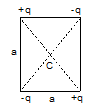JEE Main Mock Test 3 - Question 27

An LCR circuit is equivalent to a damped pendulum. In an LCR circuit the capacitor is charged to Q0 and then connected to the L and R as shown.If a student plots graphs of the square of maximum charge Q2max on the capacitor with time (t) for two different values L1 and L2 (L1>L2) of L then which of the following represents this graph correctly? (Plots are schematic and not drawn to scale)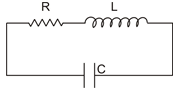JEE Main Mock Test 3 - Question 28

In the given circuit, charge Q2 on the 2µF capacitor changes as C is varied from 1µF to 3µF. Q2 as a function of ‘C’ is given properly by: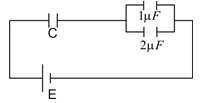JEE Main Mock Test 3 - Question 29

An inductor (L=0.03H) and a resistor (R=0.15kW) are connected in series to a battery of 15V EMF in a circuit shown. The key K1 has been kept closed for a long time. Then at t = 0, K1 is opened and key K2 is closed simultaneously. At t = 1 ms, the current in the circuit will be : (e5=150)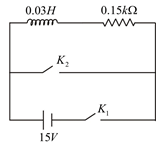JEE Main Mock Test 3 - Question 30

An example of a perfect diamagnet is a superconductor. This implies that when a superconductor is put in a magnetic field of intensity B, The magnetic field Bs inside the superconductor will be such that:

JEE Main Mock Test 3 - Question 31

A compound A and B are interconverting. Structures of A and B are shown below: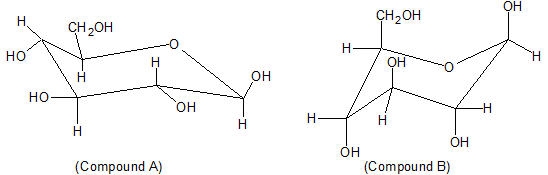Which of the following statement is correct regarding the equililbrium constant of A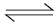B.

JEE Main Mock Test 3 - Question 32

Which of the following species is highly acidic.

JEE Main Mock Test 3 - Question 33

Which of the following statement is correct regarding the product of the following reaction?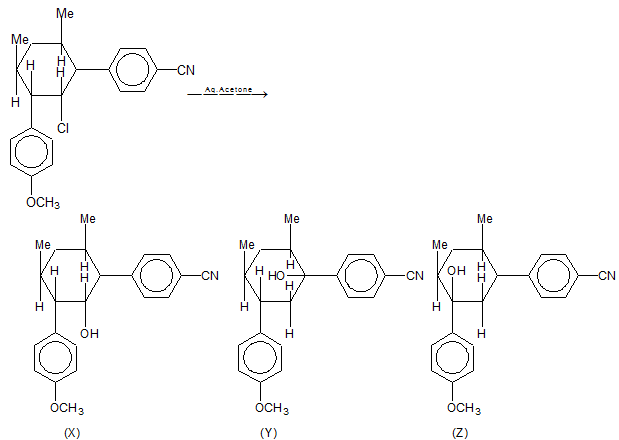JEE Main Mock Test 3 - Question 34

A face centred cubic lattice is made up of hollow spheres of X. Spheres of solid Y are present in hollow sphere X. Radius of Y is half of radius of X. What is the ratio of total volume of spheres of X unoccupied by Y in a Unit-cell and volume of unit cell.

JEE Main Mock Test 3 - Question 35

[HXeO4]- + OH- → [A] +[B] + O2 + H2O.

The products in [A] and [B] in unbalanced reaction are:

JEE Main Mock Test 3 - Question 36

B(OH)3 + NaOHNa[B(OH)4] (aq)

Addition of compound X makes the reaction to proceed in forward direction, compound X is:

JEE Main Mock Test 3 - Question 37

During the transition of an electron from n = n level to n = 1 level in H (Hydrogen), D (Deuterium) and T (Tritium) if the wavelengths of emitted photons are λH, λD and λT. Then which of the following statement is correct?

JEE Main Mock Test 3 - Question 38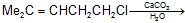Major product

Major product is:

JEE Main Mock Test 3 - Question 39

Which of the following diatomic molecules would be stabilized by the removal of an electron?

JEE Main Mock Test 3 - Question 40

The approximate pH of a solution formed by mixing equal volumes of solution of 0.1 M sodium propanoate and 0.1 M propanoic acid (ka for propanoic acid = 1.3 × 10-5 mol dm-3) will be:

JEE Main Mock Test 3 - Question 41

A catalyst lowers the activation energy of a reaction from 20 kJ mole–1 to 10 kJ mole–1. The temperature at which the uncatalysed reaction will have the same rate as that of the catalysed at 27°C is

JEE Main Mock Test 3 - Question 42

Mercurous ion (Hg22+) has magnetic moment of

JEE Main Mock Test 3 - Question 43

The velocity of electron in the second orbit of He+ will be

JEE Main Mock Test 3 - Question 44

Correct order of size of iodine species are:

JEE Main Mock Test 3 - Question 45

20 ml of 0.2 M Al2(SO4)3 is mixed with 20 ml of 0.6 M BaCl2. Concentration of Al3+ ion in the solution will be

JEE Main Mock Test 3 - Question 46

The circulation of blood in human body supplies O2 and releases CO2. the concentration of O2 and CO2 is variable but on an average, 100 ml blood contains 0.02 g of O2 and 0.08 g of CO­2. The volume of O2 and CO2 at 1 atm and at body temperature 37oC, assuming 10 lt blood in human body, is

JEE Main Mock Test 3 - Question 47

A 3 mol sample of a gas having= 1.33 at 300 K is allowed to expand under reversible adiabatic condition from 5 L to 40 L. The value ofH is

JEE Main Mock Test 3 - Question 48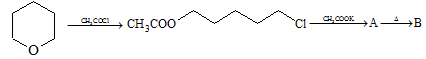JEE Main Mock Test 3 - Question 49

Aqueous solution of a salt (Y) is alkaline to litmus. On strong heating, it swells-up to give a glassy material. When conc. H2SO4 is added to a hot concentrated solution of (Y), white crystals of a weak acid separates out. Hence, the compound (Y) is

JEE Main Mock Test 3 - Question 50

A white powder when strongly heated gives off brown fumes. A solution of this powder gives a yellow precipitate with a solution of KI. When a solution of barium chloride is added to a solution of powder, a white precipitate results. This white powder may be

JEE Main Mock Test 3 - Question 51

Which nitrogen in the following compound has least pKb value?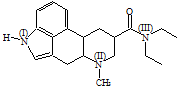JEE Main Mock Test 3 - Question 52

The slag obtained during the extraction of copper pyrites is composed mainly of

JEE Main Mock Test 3 - Question 53

Which of the following is an example of basic dye?

JEE Main Mock Test 3 - Question 54

Which of the following is a natural fibre?

JEE Main Mock Test 3 - Question 55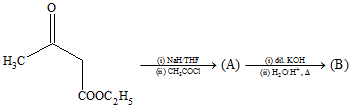The product (B) in the above sequence of reaction is

JEE Main Mock Test 3 - Question 56

Chlorination of toluene in presence of light and heat followed by treatment with aqueous NaOH gives

JEE Main Mock Test 3 - Question 57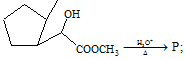'P' as a major product must be,

JEE Main Mock Test 3 - Question 58

The reaction A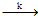Product, is zero order while the reaction BProduct, is first order reaction. For what initial concentration of A are the half lives of the two reactions equal? (Rate constant value are same for both two reaction).

JEE Main Mock Test 3 - Question 59

Two substances A and B are present such that [A0] = 4[B0] and half-life of A is 5 minutes and that of B is 15 minutes. If they start decaying at the same time following first order kinetics how much time later will the concentration of both of them would be same?

JEE Main Mock Test 3 - Question 60

40% of a mixture of 0.2 mol of N2 and 0.6 mol of H2 react to give NH3 according to the equation,N2(g) + 3H2(g) → 2NH3(g), at constant  temperature  and pressure. Then the ratio of the final volume to the initial volume of gases are

JEE Main Mock Test 3 - Question 61

Equation of the bisector of the acute angle between lines 3x + 4y + 5 = 0 and 12x – 5y – 7 = 0 is

JEE Main Mock Test 3 - Question 62

If α and β are the roots of the equation x2 - 2x + 4 = 0, then the value of αn + βn will be

JEE Main Mock Test 3 - Question 63

If A =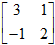and I =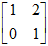, then the correct statement is

JEE Main Mock Test 3 - Question 64

The value of the determinant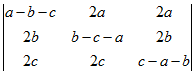will be

JEE Main Mock Test 3 - Question 65

If AM and HM between two numbers are 27 and 12 respectively, then their GM is

JEE Main Mock Test 3 - Question 66

A bag contains 3 white and 5 black balls. One ball is drawn at random. Then, the probability that it is white, is

JEE Main Mock Test 3 - Question 67

a. (b × c) = 0, then the correct statement is

JEE Main Mock Test 3 - Question 68

If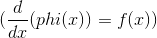, then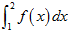is equal to

JEE Main Mock Test 3 - Question 69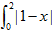dx is equal to

JEE Main Mock Test 3 - Question 70

The function sin x + cos x is maximum when x is equal to

JEE Main Mock Test 3 - Question 71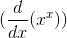is equal to

JEE Main Mock Test 3 - Question 72

The set A={ x : x ε R, x2 = 16 and 2x = 6 } equals

JEE Main Mock Test 3 - Question 73

In how many ways can 5 prizes be distributed among four students when every student can take one or more prizes?

JEE Main Mock Test 3 - Question 74

The value of 7 log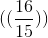+ 5 log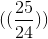+ 3 log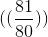is equal to

JEE Main Mock Test 3 - Question 75

The value of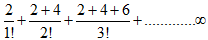is

JEE Main Mock Test 3 - Question 76

If the domain of the function f (x) = x2 - 6x + 7 is (-∞ , ∞) , then the range of function is

JEE Main Mock Test 3 - Question 77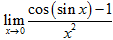is equal to

JEE Main Mock Test 3 - Question 78

In order that the function f (x) = (x + 1)1/x is continuous at x = 0,f (0) must be defined as

JEE Main Mock Test 3 - Question 79

The function f (x) = |x| at x = 0 is

JEE Main Mock Test 3 - Question 80

The function  f (x) = x1/x is

JEE Main Mock Test 3 - Question 81

The order and degree of the differential equation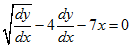are

JEE Main Mock Test 3 - Question 82

The line x + y = 4 divides the line joining the points (-1,1) and (5,7) in the ratio

JEE Main Mock Test 3 - Question 83

The angle between the pair of lines given by equation x2 + 2xy – y2 = 0, is

JEE Main Mock Test 3 - Question 84

The length of tangent from point (5,1) to the circle x2 + y2 + 6x - 4y -3 = 0 is

JEE Main Mock Test 3 - Question 85

The radius of the director circle of the hyperbola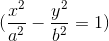is

JEE Main Mock Test 3 - Question 86

If projection of any line on coordinate axes are 3, 4 and 5, then its length is

JEE Main Mock Test 3 - Question 87

If tanθ = (1/2) and tan= (1/3) ,then the value of θ +is

JEE Main Mock Test 3 - Question 88

The principle value of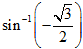is

JEE Main Mock Test 3 - Question 89

A ladder rests against a wall so that its top touches the roof of the house. If the ladder makes an angle of 60° with the horizontal and height of the house be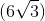, then the length of the ladder is

JEE Main Mock Test 3 - Question 90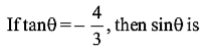Use Code STAYHOME200 and get INR 200 additional OFF Use Coupon Code
Information about JEE Main Mock Test 3 Page
In this test you can find the Exam questions for JEE Main Mock Test 3 solved & explained in the simplest way possible. Besides giving Questions and answers for JEE Main Mock Test 3 , EduRev gives you an ample number of Online tests for practice